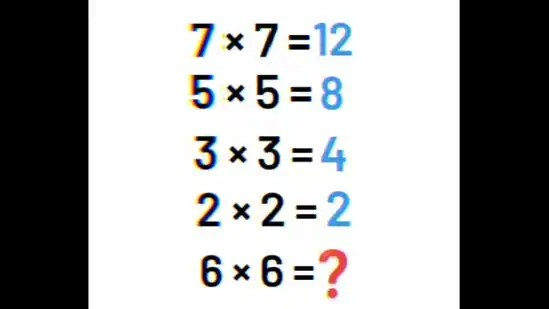Search for:
• Home/
• Editor's Pick/
• ‘99% will fail’: Can you find the answer to this maths brain teaser? | Trending## ‘99% will fail’: Can you find the answer to this maths brain teaser? | Trending

A brain teaser has been doing the rounds on Instagram with the claim that 99% of people will not be able to solve it correctly. Intriguing, right? The brain teaser features the products of a few numbers, and using that information, you need to determine the product of two other numbers.Brain Teaser: Can you find the product of the given numbers?(Instagram/@mathcince)

“99% will fail. Can you solve this?” reads the caption to this brain teaser shared on the Instagram page that goes by ‘Mathematics’. According to the brain teaser, 7 multiplied by 7 equals 12, 5 multiplied by 5 equals 8, 3 multiplied by 3 equals 4, and 2 multiplied by 2 equals 2. The question then asks what 6 multiplied by 6 will equal. Can you figure it out?

## Take a look at this maths brain teaser below:

The brain teaser was shared two days ago on Instagram. Nearly 1,500 people have liked the brain teaser, and a few of them even solved it and shared their answers in the comments section.

## Here’s how Instagram users reacted to this brain teaser:

An individual simply wrote, “Ans: 10.”

“The answer is 10 as, because don’t do multiplication, just do addition of the no. And subtract from 2. Try it,” suggested another.

A third commented, “2×2=2. 3×3=4. 4×4=6. 5×5=8. 6×6=10. 7×7=12.”

“36 is true because all on top is false,” joined a fourth.

A fifth shared, “10 is the correct answer.”

Were you able to solve this maths brain teaser? If yes, what answer did you get?